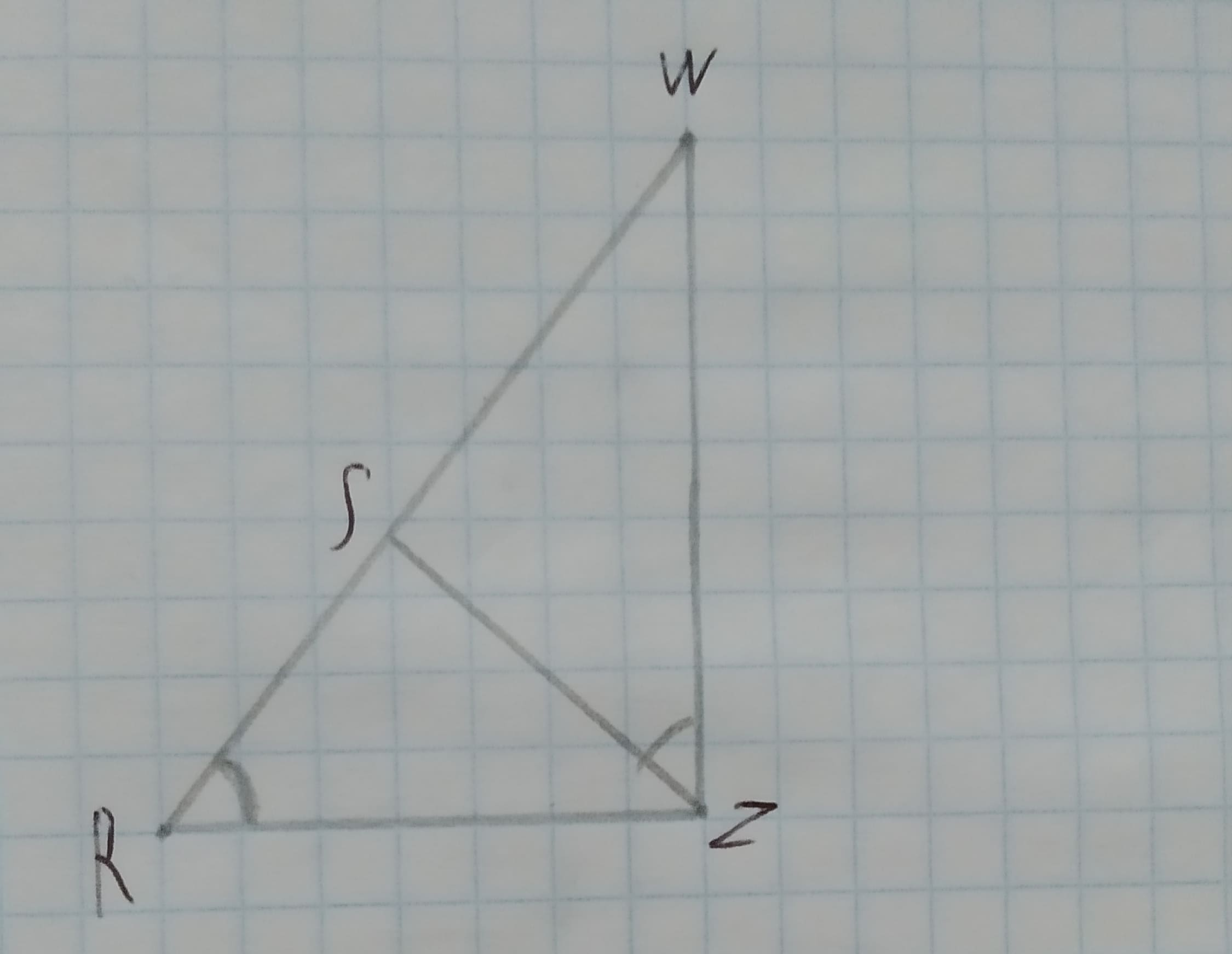# To Complete:the statement WZ=? and RS=? in the figure shown.Given:Figure is shown below.12210202811.jpgRW=15, ZR=10 and ZS=8Reeves 2021-02-02 Answered

To Complete:the statement WZ=? and RS=? in the figure shown.
Given:
Figure is shown below.RW=15, ZR=10 and ZS=8

You can still ask an expert for help

• Questions are typically answered in as fast as 30 minutes

Solve your problem for the price of one coffee

• Math expert for every subject
• Pay only if we can solve itkrolaniaN
Calculation:
In $\mathrm{△}RWZ\phantom{\rule{1em}{0ex}}\text{and}\phantom{\rule{1em}{0ex}}\mathrm{△}ZWS$.
$\mathrm{\angle }WRZ\stackrel{\sim }{=}\mathrm{\angle }WZS\therefore$(Given)
$\mathrm{\angle }W\stackrel{\sim }{=}\mathrm{\angle }W\therefore$(Common)
$WZ\stackrel{\sim }{=}WZ\therefore$(Common)
By AAS similarity, $\mathrm{△}RWZ\sim \mathrm{△}ZWS$.
Therefore,
$\frac{RW}{ZW}=\frac{ZR}{SZ}=\frac{WZ}{WS}$
$\frac{15}{ZW}=\frac{10}{8}$
$ZW=\frac{15×8}{10}$
ZW = 12
$\frac{ZR}{SZ}=\frac{WZ}{WS}$
$\frac{10}{8}=\frac{12}{WS}$
$WS=\frac{12×8}{10}$
WS=9.6
And
RS=RW-SW
RS=15-9.6
RS=5.4
Therefore, the answer is 12 and 5.4.